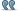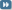• # Lineer Cebir Sorularım , Mühendislik Öğrencisi Yardımlarınızı Bekliyorum

1. ##Lineer Cebir Sorularım , Mühendislik Öğrencisi Yardımlarınızı Bekliyorum

İzmir'de Bilgisayar Mühendisliğinde okuyorum.Lineer Cebir Ödevi verildi hocamız tarafından , ilk defa zorlandım 1.soruyu kolaylıkla yaptım ama 2,3,4,5.sorularda takıldım.Yardımlarınızı bekliyorum.Şimdiden Teşekkür ederim,saygılarımla..

----------------------------------------------------------------------------------

Q1. Determine whether the below given set V is closed under the usual matrix addition and usual scalar multiplication. V is the set of all 2x2 real matrices where a=d.
The (usual) scalar multiplication: .
(The Problem 4 in Exercises 6.1, Page 278 of the text book by Kolman-Hill.)

A.1

For Matrix Addition ; Where a=d = a and d are always equal , so all 2x2 real matrices must be closed for matrix addition.Therefore , according to matrix addition it is closed under the usual matrix addition.

For Matrix Multiplication ; where a=d = , A and D are equal ( , so any k constant multiply with the matrices , its result will be same for a and d.Therefore it is always closed for scalar multiplication.

Q2. Determine whether or not the set of 3-dimensional real vectors together with the following vector addition and scalar multiplication operation constitutes a linear (vector) space over the real number set as the scalar field.
Vector addition: =. Scalar multiplication: :
A.2
For Vector Addition ; = X1,X2 and X3 are element of the vector (V).Y1 , Y2 and Y3 are scalar (F).Their result are gathering in R3 . R3 (3-dimensional real vector space )

if X1 and Y1 can be added and can be seen in the vector space.This matrix can be defined in R3 (R3 which means that it has there rows and it is 3-dimensional real vector space). As a result , The result of X and Y set aren’t satisfied Closureness specify.This means that , The result isn’t in a vector space according to closureness.It is not closed.

For Scalar Multiplication , : , This means that

Q3. Determine whether or not the set of all positive real numbers u with the vector addition defined as u+v= uv and scalar multiplication defined as c·u= constitutes a linear (vector) space over the real number set as the scalar field. If it is not a vector space, list the properties that fail to hold.
(The Problem 17 in Exercises 6.1, Page 278 of the text book by Kolman-Hill.)
Where, uv denotes the real multiplication and is the c’th power of u. Note that u+v is not the usual real addition: Use any other symbol instead of + if you feel uncomfortable with +.

Q4. Consider the unit square shown in the accompanying figure. Let W be the set of all vectors of the form , where 0≤x≤1, 0≤y≤1. That is the set of all vectors whose tail is at the origin and whose head is a point inside or on the square. Is W a subspace of R2? Explain.
(The Problem 4 in Exercises 6.2, Page 287 of the text book by Kolman-Hill.)

Q5. Which of the following subset of P2 (i.e. the set of second order real polynomials) are subspaces? The set of all polynomials of the form
a) a2t2+a1t+a0 where a1= a0=0. b) a2t2+a1t+a0 where a1= 2 a0. c) a2t2+a1t+a0 where a2+a1+a0=02. İlk önce türkçeye çevirmek lazım tabi.3.Admin'den alıntıİlk önce türkçeye çevirmek lazım tabi.

Efendim Çok sıkışık durumdayım , bütün notlarım tam , Lineer Cebir dersinde Determinantlara kadar hep iyi notlar aldım , Nezaman Vektörlere geldik batırmaya başladım , sizin verceğiniz cevaplar benimkonuyu anlamamada yardımcı olucak.Size çok dua edicem , Anlmadığınız yerleri söyleyin ben türkçeye çeviririm efendim size.4. 1.Soruyu yapmanıza gerek yok ben hallettim efendim

SORULARIN TÜRKÇELERİ BURDA MEVCUTTUR TEKRAR TEŞEKKÜR EDERİM , ACİL OLARAK CUMA GÜNÜ VERMEM GEREK LÜTFEN YARDIM EDİN.

2.Soru
Ilk sekılde yanı 5 tane ozellıgı tek tek kontrol edeceksın ,scalar multıplıcatıon
da ıse 5 olan sm ozellıklerını tektek kullanarak bunun lınear space olup olmadıgını
kontrol edeceksın

3.soru
Burada bızden normal olan toplama ıslemını u+v yerıne uv seklınde tanımlayarak
Toplama ıslemının 5 ozellıgını saglayıp saglamadıgını kontrol etmemızı sonra 5 tane ca
Maya gore kontrol etmemızı ıstıyo sonra uc yide scalar multıplıcatıona gore kontrol
Etmemız ıstenıyor

4.soru
Bizim rkare deki bu sekılın ıcındekı 0 1 arasındakı x ve 0 bır arasındakı y nın altuzay olup olmadıgını
Kontrol etmemıızı ıstıyor
5.soru
Verılen polınomların subspace ( alt uzay ) olup olmadıgını tek tek gostermemizi istiyor bizden.5. Kötü bir tercüme yapmışsınız. Birkaç çözüm yazabilirim.

Q4 için:
u,v∈W olacak şekilde u=(1/2,1/2) ve v=(1,1) seçelim. Allt uzay tanımı gereği toplamaya göre kapalı olmalıdır. u+v=(3/2,3/2)∉W olduğundan W, R2'nin alt vektör uzayı değildir.

Q3 için:6. Hocam ellerinize sağlık. Bunlarıda anlayıp çözebildiniz ya süpersiniz.7. Hocam 1 ve 2 'yi kendim yaptım , 3 ve 4'ü sizin yaptığınızdan faydanalarak o mantığa göre tamamen kendim yaptım ve bitek 5.Sorum kalmıştı , onuda a ve c şıklarını bi arkadaştan aldım , b şıkkını yapmadan gönderdim.Tüm yardımlarınız için teşekkür ederim sayenizde8.Admin'den alıntıHocam ellerinize sağlık. Bunlarıda anlayıp çözebildiniz ya süpersiniz.
Estağfurullah, Estağfurullah...

5. souda da iki polinom alıp toplamına ve çarpımına bakcaksınız verilen şartlar çerçevesinde zaten.1. Foruma üye olmana gerek yok! Facebook hesabınla yorumlarını bekliyoruz!Forum Kullanım ve Gizlilik Kuralları